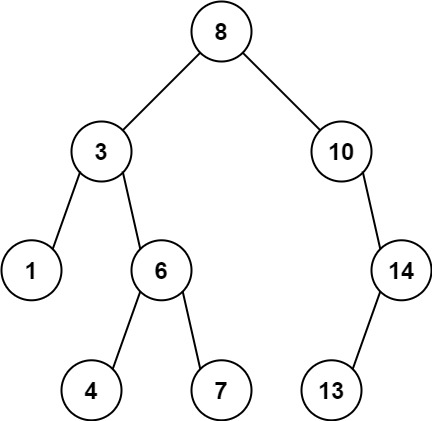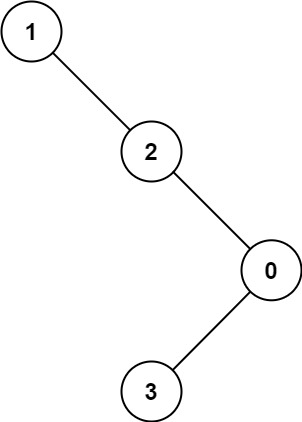# 1026. Maximum Difference Between Node and Ancestor

Maximum Difference Between Node and Ancestor - LeetCode

Given the root of a binary tree, find the maximum value v for which there exist different nodes a and b where v = |a.val - b.val| and a is an ancestor of b. A node a is an ancestor of b if either: any child of a is equal to b or any child of a is an ancestor of b.

Example 1:Input: root = [8,3,10,1,6,null,14,null,null,4,7,13] Output: 7 Explanation: We have various ancestor-node differences, some of which are given below : |8 - 3| = 5 |3 - 7| = 4 |8 - 1| = 7 |10 - 13| = 3 Among all possible differences, the maximum value of 7 is obtained by |8 - 1| = 7.

Example 2:Input: root = [1,null,2,null,0,3] Output: 3

Constraints:

``````The number of nodes in the tree is in the range [2, 5000].
0 <= Node.val <= 105
``````

• code
``````# Definition for a binary tree node.
# class TreeNode:
#     def __init__(self, val=0, left=None, right=None):
#         self.val = val
#         self.left = left
#         self.right = right
class Solution:
def maxAncestorDiff(self, root: Optional[TreeNode]) -> int:
def rec(root):
if not root:
return (inf, -inf, 0)
lmini, lmaxx, lmaxdiff = rec(root.left)
rmini, rmaxx, rmaxdiff = rec(root.right)

curmaxdiff = -inf
if root.left:
curmaxdiff = max(curmaxdiff, abs(root.val - lmini), abs(root.val - lmaxx))
if root.right:
curmaxdiff = max(curmaxdiff, abs(root.val - rmini), abs(root.val - rmaxx))

mini = min(lmini, rmini, root.val)
maxx = max(lmaxx, rmaxx, root.val)
maxdiff = max(lmaxdiff, rmaxdiff, curmaxdiff)
return (mini, maxx, maxdiff)

return rec(root)

``````
• code
``````class Solution:
def maxAncestorDiff(self, root: Optional[TreeNode]) -> int:
def rec(root, curmin, curmax):
if not root:
return curmax - curmin
curmin = min(curmin, root.val)
curmax = max(curmax, root.val)
return max(rec(root.left, curmin, curmax), rec(root.right, curmin, curmax))

return rec(root, root.val, root.val)

``````